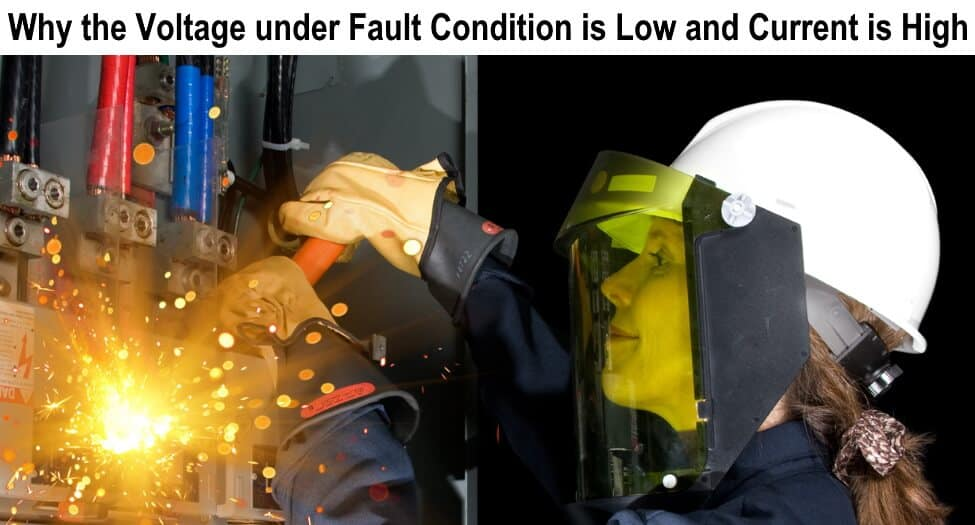# Why the Reactance & Voltage under Fault Condition is Low and Current is High

Why the Voltage and Reactance of a system under fault condition is low and fault current may rise to the highest dangerous value?

We know that the power in watts is constant from supply source and under fault conditions in ac circuits such as short circuit there is no load and impedance “Z”, reactance i.e. inductive reactance and capacitive reactance which is equal to the overall resistance of ac circuits.When short circuit occurs, there will be no resistance or reactance and current will be too much high. In this case, when power is constant and current increases, voltage will be decreased.

To understand this basic point, let consider the following example.

Suppose, (In normal condition)

Power = P = 1000 Watts

Voltage = V = 110 Volts

Current = I = 9.09 Amp.

But in Short circuit condition, (When current is too high)

Then,

P = 1000 Watts

I = 1000 A

V = P / I

1000 Watts /1000A = 1 V.

To know why the system voltage under fault condition is too low, according to the ohm’s law:

V = I x R

As in case of short circuit, there will be no load i.e. resistance and reactances, so if we put resistance as zero in ohm’s law, the voltage will be too low due to high value of current flowing through the conductor as the circuit resistance is almost zero.

So we can say that, in case of short circuit and fault conditions, Inductive reactance is zero, current increases to the higher value and voltage decreases as power is constant from the power house.

Good to know:

• The system reactances ( Inductive reactance and capacitive reactance) is almost zero under fault conditions such as short circuit.
• In case of short circuit, the system resistance is ideally zero.
• The value of voltage between two points is theoretically when short circuit occurs.
• Current rise to the dangerous value in case of short circuit which leads to damage the connected appliances and installation.